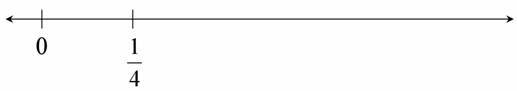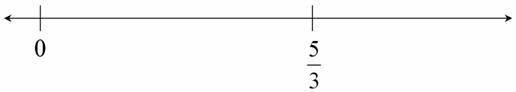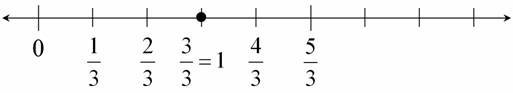# Find 1

Alignments to Content Standards: 3.NF.A.2

1. Locate 1 on the number line. Label the point. Be as exact as possible.2. Locate 1 on the number line. Label the point. Be as exact as possible.## IM Commentary

The purpose of this task is to assess whether students understand fractions as being built from unit fractions and whether they can accurately locate fractions on the number line. This task could also be used in an instructional setting where students work in pairs or small groups to try to figure out how to use the given information to locate other numbers on the number line. If teachers give this task to students without scaffolding, it allows them an opportunity to engage in MP 1, Make sense of problems and persevere in solving them. This task includes the seeds of several important ideas.

Typically, students start with 0 and 1 on the number line and find unit (and other) fractions. In part (a) students must work in the other direction: they use a unit fraction to find 1 on the number line. This task reinforces the idea that a point on the number line represents a number. This kind of work also lays the groundwork for students to represent addition and subtraction on the number line in grade 4.

Part (b) reinforces the meaning of the numerator and the denominator. When students begin with the interval from 0 to 1, they typically start by partitioning that interval into unit fractions defined by the denominator of the fraction they are given. Here they must start with the numerator because they are partitioning the interval between 0 and 5/3. This part also helps reinforce the notion that when a fraction has a numerator that is larger than the denominator, it has a value greater than 1 on the number line.

The following lists related tasks in order of sophistication:

• Locating Fractions Less than One on the Number Line
• Locating Fractions Greater than One on the Number Line
• Closest to $\frac12$
• Find 1
• Find $\frac23$
• Which is Closer to 1?

To see an annotated version of this and other Illustrative Mathematics tasks as well as other Common Core aligned resources, visit Achieve the Core.

## Solution

While it is not necessary to name all of the intervals on the number line, we expect many students will do so.

1. There are 4 fourths in 1, so if we take the length from 0 to $\frac14$ four times, we will find 1.2. $\frac53$ is 5 equal pieces where 3 pieces make 1. So if we partition the interval between 0 and $\frac53$ into 5 pieces, each will be a thirdand 3 thirds is 1.